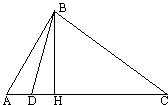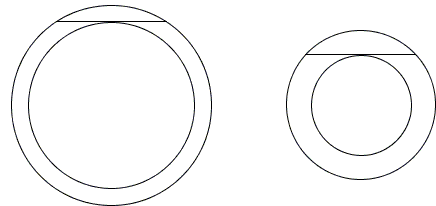# Sample Corollaries from the Pythagorean Theorem

The Pythagorean Theorem plays an important role in geometry and in mathematics in general. On this page I'll try to collect several statements some of whose proofs depend on the Pythagorean Theorem.

### The Arithmetic - Geometric Means InequalityFor positive $a$ and $b,$ $(a + b)/2 ≥ \sqrt{ab},$ with equality iff $a = b.$

For a proof, assume $a > b$ and construct a right triangle with hypotenuse $(a+b)/2$ and one side equal to $(a-b)/2.$ From the Pythagorean Theorem, the remaining side will equal $\sqrt{ab}.$ Since, in a right triangle, the hypotenuse is the largest side, the inequality has been proved in the case $a > b.$ It obviously turns into equality when $a = b.$

One may argue that the proof followed from an algebraic identity

$(a + b)^{2} - (a - b)^{2} = 4ab.$

In that case, the Pythagorean Theorem furnishes an intuitive geometric illustration. Just draw two touching circles with radii $a/2$ and $b/2$ as in the diagram.

$(a + b)/2$ is known as the arithmetic mean of the numbers $a$ and $b;$ $\sqrt{ab}$ is their geometric mean also known as the mean proportional because if $k = \sqrt{ab}$ then $a/k = k/b$ and vice versa.As in the case Isoperimetric Inequality, the AM-GM inequality also allows for two equivalent extremal problems:

1. Among all pairs of numbers with a given product find two whose sum is minimal.
2. Among all pairs of numbers with a given sum find two whose product is maximal.In both cases, the extremal value is attained when the two numbers coincide. The latter fact has a nice geometric illustration which also suggests another proof for the Arithmetic Mean - Geometric Mean Inequality.

The former is often rewritten in a different form:

(1)

For $x > 0,$ $x + 1/x \ge 2,$ with equality iff $x = 1.$

The Arithmetic Mean - Geometric Mean Inequality for sequences of numbers was first proven when the length of the sequence was a power of $2$ and from here for an arbitrary integer. (1) also extends for an arbitrary number of positive numbers:

Let $x_{i} > 0,$ $i = 1,2,\ldots ,n.$ Then $x_{1}/x_{2} + x_{2}/x_{3} + \ldots + x_{n}/x_{1} \ge n.$

Actually more is true. For positive $x_{i}$'s, let p be an arbitrary permutation of the set of indices $\{1,\ldots ,n\}.$ Then

$x_{1}/x_{p(1)} + x_{2}/x_{p(2)} + \ldots + x_{n}/x_{p(n)} \ge n.$### Cosine Rule

The Cosine Rule is an obvious generalization of the Pythagorean Theorem. However, its variant that does not use trigonometric functions is a direct consequence of the latter.#### Lemma

The difference of squares of two sides of a triangle equals the difference of squares of their projections on the third side:

(2)

$AB^{2} - BC^{2} = AH^{2} - CH^{2}.$

For a proof, use Pythagoras' Theorem twice: $AB^{2}=AH^{2}+BH^{2}$ and $BC^{2}=CH^{2}+BH^{2}.$ Subtract one equation from the other.

From (2), $BC^{2} = AB^{2} + CH^{2} - AH^{2}.$ If $ABC$ is an acute triangle then $CH = AC - AH$ which gives

(3.1)

$BC^{2} = AB^{2} + AC^{2} - 2\cdot AC\cdot AH$If angle $A$ is obtuse, then $CH = AC + AH$ and (2) yields

(3.2)

$BC^{2} = AB^{2} + AC^{2} + 2\cdot AC\cdot AH$### Locus of a point

Given points $A$ and $C$. Find the locus of a point $B$ for which the difference of the squares of the distances to $A$ and $C$ is constant.

The lemma above gives an immediate solution to this problem. Let point $H$ on $AC$ be such $AH^{2} - CH^{2}=K,$ where $K$ is a given constant. Then, as (2) shows, $AB^{2} - BC^{2} = K,$ for any $B$ on the line perpendicular to $AC$ through $H.$

Different lines correspond to different constants $K.$ It is practically immediate that the location of $H$ depends linearly on $K$ so that the correspondence between $H$ and $K$ is 1-1 and onto.

Given two circles $(O)$ and $(o)$ with radii $R$ and $r$ respectively. Points $X$ on the radical axis satisfy $OX^{2}-R^{2}=oX^{2}-r^{2}.$ In other words, $OX^{2}-oX^{2}=R^{2}-r^{2}=\mbox{const}.$ The result we just obtained could be reformulated as the following:

Let $\mathcal{O}(X)$ be the power of point $X$ with respect to $(O),$ $\mathcal{o}(X)$ power of point $X$ with respect to $(o).$ Then the locus of point $X$ for which $\mathcal{O}(X)-\mathcal{o}(X)$ is constant is a line parallel to the radical axis of the two circles.### Stewart's Theorem

Coxeter and Greitzer remark that the theorem below was named after M.Stewart, who stated it in 1746, but was probably discovered by Archimedes about 300 B.C. However, the first known proof is by R.Simson, 1751.Let point $D$ lie between the vertices $A$ and $C$ of $\Delta ABC.$ Then

$AB^{2}\cdot DC + BC^{2}\cdot AD - BD^{2}\cdot AC = AC\cdot DC\cdot AD.$

For the definiteness sake, let $H$ (the foot of the altitude $BH)$ lie between $D$ and $C,$ as in the diagram. Apply (3.1) to $\Delta BCD$ and (3.2) to $\Delta ABD:$

$BC^{2} = BD^{2} + DC^{2} - 2\cdot DC\cdot DH,\\ AB^{2} = BD^{2} + AD^{2} + 2\cdot AD\cdot DH.$

Multiply the first identity by $AD,$ the second by $DC,$ and add the two

\begin{align} BC^{2}\cdot AD + AB^{2}\cdot DC &= BD^{2}\cdot (AD + DC) + DC^{2}\cdot AD + AD^{2}\cdot DC\\ &= BD^{2}\cdot AC + AD\cdot DC\cdot AC. \end{align}### Medians

Let $D$ be the midpoint between $A$ and $C.$ As usual, let $a = |BC|,$ $b = |AC|,$ $c = |AB|,$ and $m_{b} = |BD|.$ After division by $a,$ Stewart's Theorem then takes the form

$m_{b}^{2} = (a^{2} + c^{2})/2 - b^{2}/4.$### Altitudes and Heron's Formula

Note that (3.1) applies to an acute while (3.2) to an obtuse angle. Since, in a triangle, only one angle may be obtuse, (3.1) is always applicable. Let, for example, angle A be acute. Then from (3.1)

$a^{2} = b^{2} + c^{2} - 2b\cdot AH$

On the other hand, in the right triangle $AHB,$ $BH^{2} = c^{2} - AH^{2}$ which, combined with the previous identity, yields

\begin{align}BH^{2} &= c^{2} - (b^{2} + c^{2} - a^{2})^{2}/(4b^{2})\\ &= (c - (b^{2} + c^{2} - a^{2})/(2b))(c + (b^{2} + c^{2} - a^{2})/(2b))\\ &= (2bc - b^{2} - c^{2} + a^{2})(2bc + b^{2} + c^{2} - a^{2})/(4b^{2})\\ &= (a^{2} - (b - c)^{2})((b + c)^{2} - a^{2})/(4b^{2})\\ &= (b - a + c)(b + a - c)(a + c - b)(a + b + c)/(4b^{2}) \end{align}

Introduce the semiperimeter $p = (a + b + c)/2.$ Then

$BH^{2} = 4p(p - a)(p - b)(p - c)/b^{2}$

Which is just another way of writing Heron's formula. Thus, the two facts: Pythagoras' Theorem and Heron's formula each have an independent proof. But, in addition, each can be derived from the other.

### Remark

Dr. S. Brodie has kindly prepared a demonstration of how Pythagoras' Theorem is used to construct a regular pentagon. He has also observed that the Theorem is equivalent to the famous Parallel (or Fifth) Postulate.

Finally, there is an interesting, only recently discovered, application of the theorem and an interesting puzzle the theorem helps to solve.### Area of a Ring

The area of a circle depends on a single parameter: its radius. For radius $R,$ it equals $\pi R^{2}.$ Curiously, the area of a circular ring (annulus), i.e., the shape between two concentric circles, also depends on a single parameter [Rohrer, #25].The parameter is the length (or, more conveniently, its half-length) of the chord of the outer circle tangent to the inner circle. If a denotes half the chord in question, the area of the circular ring is given by $\pi a^{2}.$ To see why this is so draw the radii of the two circles to complete a right triangle. Apply the Pythagorean theorem.It may be argued independent of the Pythagorean theorem that the area swept by a fixed length segment is the same whether it rotates around one of its points or slides as tangent to a given curve. Establishing this result then will furnish an additional proof of the Pythagorean theorem.

### References

1. H. S. M. Coxeter, S. L. Greitzer, Geometry Revisited, MAA, 1967
2. R. B. Nelsen, Proofs Without Words, MAA, 1993
3. D. Rohrer, More Thought Provokers, Key Curriculum Press, 1994